# Overview

Clustering is an unsupervised learning technique widely used in several disciplines, such as machine learning, bioinformatics, image analysis or pattern recognition. Gene expression data clustering – as well as in other omic disciplines – is useful to find groups of genes with similar behavior and may provide very useful information for the understanding of certain biological processes.

This clustering of gene expression data can be performed onto genes, samples or over the time variable. In the last case, called ‘time series genes expression’, it is possible to identify genes with similar conduct and obtain sets of responses to certain situations. This has allowed to highlight environmental stress response, circadian rhythms pathway, treatment response, etc.

Despite the fact that there are several generic R packages for the analysis of clustering time series – kmlShape , dtwclust, clue, TSclust –, there is no a single algorithm that satisfactorily solves all problems and each clustering problem requires a specific approach. There are other specific packages to clustering time series in gene expression which seek minimize the high sensitivity to noise of other generic algorithms – TSMixclust, ctsGE, MFuzz – . MFuzz minimizes the high noise sensitivity of other algorithms through a fuzzy clustering, on the other hand TSMixclust is a soft-clustering that uses mixed-effects models with non-parametric smoothing spline fitting. ctsGE seeks to minimize noisy data in two steps, first define groups based on their expression profiles (expression index) and then, genes with same index are clustered by applying kmeans. Despite the fact that these methods attempt to solve the important task of minimizing the impact of the noise on clustering, genes with similar expression – in magnitude – typically end up in the same groups, even though they have different temporal evolutions.

Occasionally, however, the researcher’s main interest lies in finding genes with similar patterns (similar trajectories) although these occur at different levels of expression. Therefore, genes with a similar temporal evolution are therefore sought regardless of their magnitude of expression.

Let us suppose that we subject an individual to a treatment and measure the expression of three (or ten thousand) of his genes at the time the treatment begins, at one week, two weeks and three weeks after (Fig. 1).

The lines in figure 1 (a,b,c) represent 3 tracks (e.g. the expression of 3 genes at 4 different times). The trajectories $$T_a$$ and $$T_c$$ are identical in terms of slopes, they only differ in the magnitude of their expression. On the other hand the track $$T_b$$ would be closer to the track $$T_a$$ than to $$T_c$$.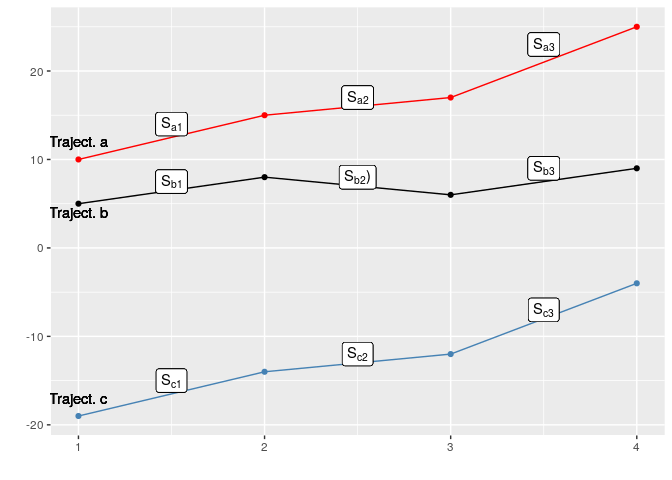Figure 1. Three different trajectories at four times to exemplify the possible relationships or classifications of the data.

Intuitively, if we had to determine the distance between these trajectories, we would think that $$T_a$$ is closer to $$T_b$$ than to $$T_c$$. In addition, $$T_c$$ is closer to $$T_b$$ than to $$T_a$$. You could quantify those distances with different metrics:

• Euclidean distance

$\begin{matrix} & a &b \\ b & 21.24 & \\ c & 54.00 & 39.41 \end{matrix}$

Other distance metrics based more specifically on time series such as Fréchet or DTW provide similar results:

• Fréchet Distance

$\begin{matrix} & a &b \\ b & 16 & \\ c & 29 & 24 \end{matrix}$

• DTW Distance

$\begin{matrix} & a & b \\ b & 42 & \\ c & 158 & 104 \end{matrix}$

However, if the main interest is to determine which trajectories have similar behaviour over time regardless of the magnitude of their expression, it is necessary to use purely geometric criteria and look for similarities based on their slopes. In this case, the distance matrix would look as follows:

$\begin{matrix} & a & b \\ b & 6.71 & \\ c & 0.00 & 6.71 \end{matrix}$ Where it can be seen that the distance between the $$a$$ and $$c$$ trajectory is zero because these are two lines with identical slopes and the distance from both to $$b$$ is the same.

Therefore, we might find a researcher interested in identifying and grouping sets of genes with similar behaviours according to different points of view:

• Genes clustered with similar levels of expression, i.e. genes whose trajectories are close in terms of physical distance (fig.2 B).

• Genes grouped with similar evolution regardless of their physical distance. In this second scenario, we are dealing with genes that respond in a similar way, but with different intensity (fig.2 C).

• It might also be interesting to group genes according to both factors (distance + tendency) (fig.2 D).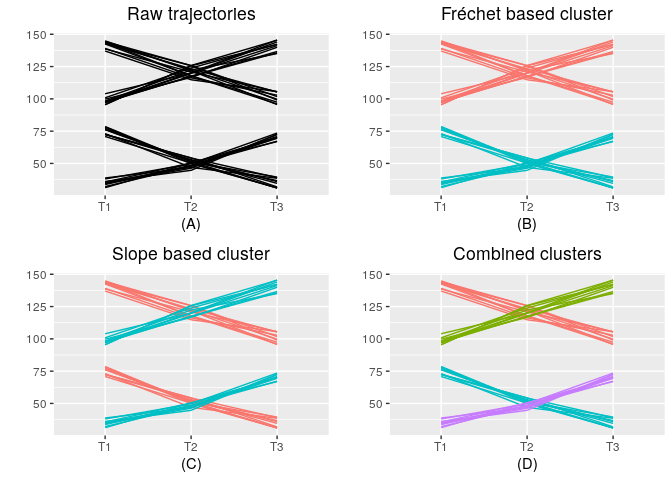Figure 2. Different possibilities of classifying the tracks from the whole data set (a) according to its Fréchet distances (b), according to its slopes (c) or a combination of both (d).

Through this package we propose a methodology that allows to group these paths based on physical distances by using distance metrics adapted to time series (Fréchet’s distance) and a new approach based on similarity of slopes between trajectories, where the trajectories are grouped according to this proximity regardless of the distance between them.

Furthermore, a combination of both classifications is available, so that the final result would be trajectories grouped according to their values and their evolutions.

Since in many studies (especially in different comic disciplines), the number of trajectories can be very large (thousands or tens of thousands), a methodology has been developed based on the existing one in the kmlShape package. By means of a pre-grouping, a series of “representatives” of the trajectories is obtained, called senators; these senators are then classified by means of some of the proposed algorithms. At last, the set of trajectories is assigned to the cluster to which its senator has been assigned. In this way the computational cost and the risk of overflowing the memory is reduced.

# Getting started

Install from Bioconductor

if (!requireNamespace("BiocManager"))
install.packages("BiocManager")
BiocManager::install("tscR")

Install the latest development version in install_github.

devtools::install_github("fpsanz/tscR")

Read the vignette (this document):

library(tscR)
browseVignettes("tscR")

# Simple clustering

## Based on slope distance

The input data has to be a dataframe or matrix where the rows will be each of the trajectories and the columns the times (Table 1).

Initially, we are going to load a set of example trajectories included in the library.

data(tscR)
df <- tscR
Table 1. First six rows from the example data matrix at three different times that have been studied for one sample.
T1 T2 T3
6.162 5.900 6.602
3.344 3.676 2.948
1.058 0.881 -0.513
4.417 6.741 7.196
2.409 3.799 2.190
1.057 1.880 -1.038

tscR includes 300 observations (trajectories) with data taken at 3 regular time-points (day 1, day 2, day 3).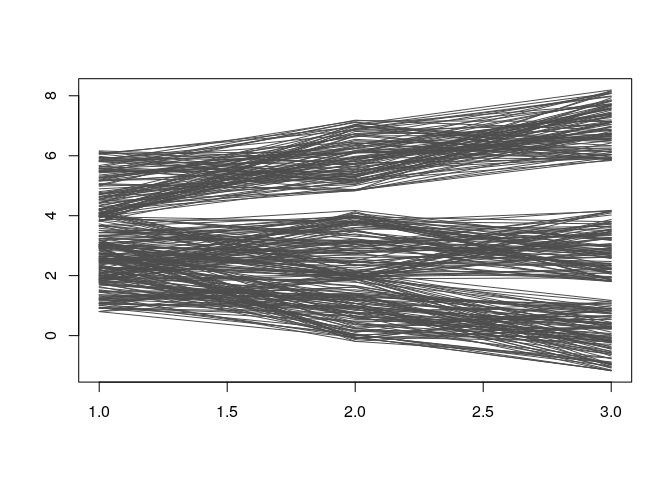Figure 3. Set ot 300 example trajectories that tscR package includes to work with.

Following this, the similarity matrix is calculated and its size will be n x n, where n represents the number of rows in the input matrix. This matrix is an object of ‘’dist’’ class and contains the similarities between trajectories based on similar slopes.

time <- c(1,2,3)
sDist <- slopeDist(df, time)

The next step would involve grouping the trajectories based on similarities in their slopes regardless of the distance between them (meter referencia al paper).

sclust <- getClusters(sDist, k = 3)

The result may be displayed with the plotCluster function. This function assumes as parameters the original data (data), the object obtained from getClusters (clust) and the clusters to be displayed: “all” to display them all in a single graphic, an integer to display the trajectories of that cluster or a vector defining which clusters should be displayed (one per subplot).

plotCluster(data = df,
clust = sclust,
ncluster = "all")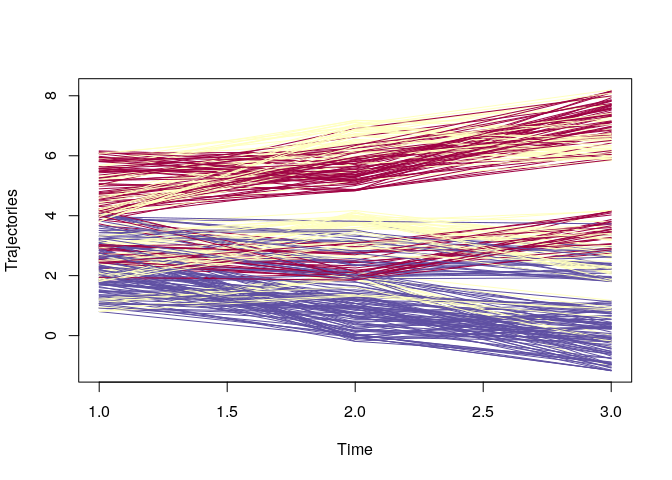plotCluster(df, sclust, 1)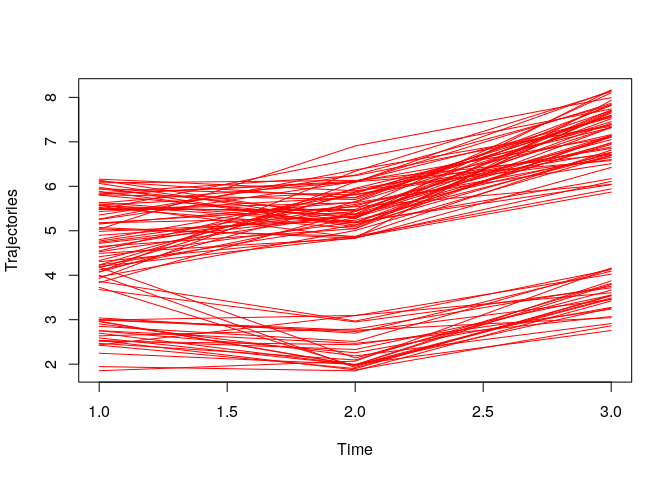plotCluster(df, sclust, c(1:2))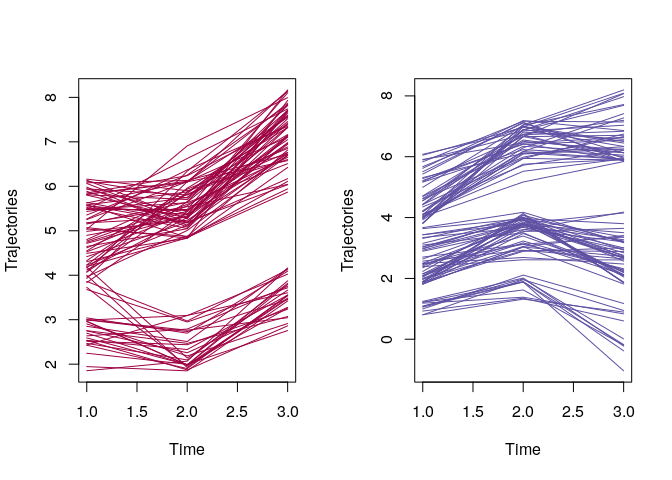As it can be appreciated in this last graph, the trajectories with descending - ascending evolution have been grouped on one side, independently of their distance (left plot) and those with ascending - descending evolution on the other side (right plot).

## Based on Fréchet distance

This is a measure of similarity between curves that takes into account the location and order of the points along the curve.

The procedure seems to be similar to the previous case:

• Calculate distance matrix.
• Get the clusters.
• Display the results.
fdist <- frechetDistC(df, time)
fclust <- getClusters(fdist, 3)
plotCluster(df, fclust, "all")